# H-block cross-validation

#### 2023-03-09

Spatial autocorrelation can severely bias transfer function performance estimates. Telford and Birks (2009) suggested h-block cross-validation as a means of obtaining unbiased transfer function estimates. The problem is to estimate the optimal value of h: too small and the performance estimates are still over-optimistic, too large and the performance estimates are pessimistic. Trachsel and Telford (2015) presented three methods to estimate h:

1. the performance of a transfer function on a spatially independent test set
2. the autocorrelation in residuals of a weighted averaging transfer function
3. Comparing the variance explained of a transfer function trained on random environmental data with similar spatial structure as the environmental data of influence with the correlation between the simulated data and the environmental data of influence.
library(palaeoSig)
library(rioja)
library(sf)
library(gstat)
library(dplyr)
library(tibble)
library(tidyr)
library(purrr)
library(ggplot2)
theme_set(theme_bw())

set.seed(42) # for reproducibility 

## 1. Spatially independent test set

We use the foraminifera dataset by Kucera et al. (2005). We split the dataset into two parts, a North Atlantic (NA) dataset (north of 3°N) and a South Atlantic (SA) data set (south of 3°S). We use the NA dataset as training set and the SA dataset as spatially independent test set.

# load data
data(Atlantic)
meta <- c("Core", "Latitude", "Longitude", "summ50")

# N Atlantic
N_Atlantic <- Atlantic |>
filter(Latitude > 3) |>
slice_sample(n = 300) # subsample for speed
N_Atlantic_meta <- N_Atlantic |>
select(one_of(meta)) |>
as.data.frame() # to keep rdist.earth happy
N_Atlantic <- N_Atlantic |>
select(-one_of(meta))

# S Atlantic
S_Atlantic <- Atlantic |>
filter(Latitude < -3)
S_Atlantic_meta <- S_Atlantic |>
select(one_of(meta))
S_Atlantic <- S_Atlantic |>
select(-one_of(meta))

## convert N_Atlatic_meta to an sf object

N_Atlantic_meta <- st_as_sf(
x = N_Atlantic_meta,
coords = c("Longitude", "Latitude"),
crs = 4326
)

# calculating distances among the sampled points in the
# North Atlantic foraminifera data set
geodist <- st_distance(N_Atlantic_meta) |>
units::set_units("km") |>
units::set_units(NULL)

# values of h for which h-block cross-validation is calculated
threshs <- c(0.01, 300, 600, 900, 1200)

# h-block cross-validation of the NA foraminifera dataset for
# different values of h
res_h <- map(threshs, function(h) {
mod <- MAT(N_Atlantic, N_Atlantic_meta$summ50, k = 5, lean = FALSE) mod <- crossval(mod, cv.method = "h-block", h.dist = geodist, h.cutoff = h) tibble( h = h, RMSE = performance(mod)$crossval["N05", "RMSE"],
R2 = performance(mod)$crossval["N05", "R2"] ) }) |> list_rbind() First, we estimate the performance of the North Atlantic (NA) foraminifera training set when applied to the spatially independent data set from the South Atlantic (SA), which in our case contains the samples used by Kucera et al. (2005) that are situated south of 3°S. # Leave-one-out cross-validated RMSEP using MAT with k = 5 round(res_h[1, "RMSE"], 2) # Predicting the South Atlantic test set mod_NA <- MAT(N_Atlantic, N_Atlantic_meta$summ50, k = 5)
pred_SA <- predict(mod_NA, newdata = S_Atlantic)$fit # Determining RMSEP of the SA test set rmse_mat <- sqrt(mean((pred_SA[, 1] - S_Atlantic_meta$summ50)^2))
# RMSEP of the SA test set using MAT with k = 5
round(rmse_mat, 2)

The RMSEP of the NA training set is 1.28 while the RMSEP of the spatially independent SA data set is somewhat larger: 2.1. This is indicative of spatial autocorrelation. We have to find the the removal distance h at which the h-block cross-validated RMSEP and the RMSEP of the SA test set are similar.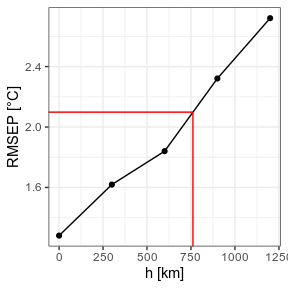Figure 1: Root mean square error of prediction (RMSEP) as a function of removal distance h. Dashed horizontal line indicates RMSEP found on a spatially independent test set.

Using linear interpolation, an h-block distance of 761 km gives a cross-validated RMSEP equivalent to the the RMSEP of a spatially independent test set.

## 2. Variogram range

The second method proposed in Trachsel and Telford is to fit a variogram to detrended residuals of a weighted average model and use the range of the variogram as h.

# WA model
modwa <- crossval(WA(sqrt(N_Atlantic), N_Atlantic_meta$summ50, mono = TRUE)) # residuals of the WA model wa_resid <- residuals(modwa, cv = TRUE) # detrend to remove edge effects (loess with span = 0.1) detrended_resid <- resid(loess(wa_resid[, 1] ~ N_Atlantic_meta$summ50,
span = 0.1
))

# variogram of the detrended residuals of the WA model
v <- variogram(detrended_resid ~ 1, data = N_Atlantic_meta)
# Fitting a spherical variogram (partial sill, range and nugget are
# approximately estimated from the empirical variogram)
vm <- fit.variogram(v, vgm(psill = 2, "Sph", range = 1500, nugget = 0.5))
plot(v, vm)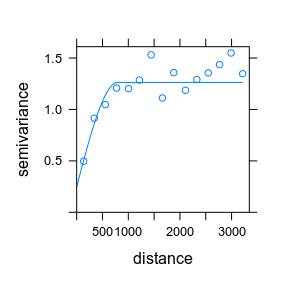Figure 2: Semi-variogram fitted to detrended residuals of a weighted averaging model.

The estimated range of a spherical variogram model fitted to the detrended residual of a WA model is 794 km.

## 3. Variance explained

The third method suggested is based on simulated environmental variables with the same spatial structure as the environmental variable of interest. The transfer function performance (r2) of the simulated variable is compared to the r2 between the environmental variable of interest and the simulated variables. The optimal value for h is the value that minimises the difference these two r2.

To simulate the environmental variables of interest, we first have to estimate their spatial structure with a variogram and then use kriging with the variogram to simulate environmental variables with same spatial structure as the observed variable.

# Estimate the variogram model for the environmental variable of interest
ve <- variogram(summ50 ~ 1, data = N_Atlantic_meta)
vem <- fit.variogram(ve, vgm(40, "Mat", 5000, .1, kappa = 1.8))
plot(ve, vem)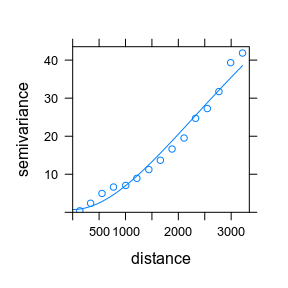Figure 3: Semi-variogram model (Matérn class) fitted to the North Atlantic summer sea temperature at 50 m depth.


# Simulating environmental variables
sim <- krige(sim ~ 1,
locations = N_Atlantic_meta,
dummy = TRUE,
nsim = 100,
beta = mean(N_Atlantic_meta$summ50), model = vem, newdata = N_Atlantic_meta ) #> [using unconditional Gaussian simulation] # convert back to a regular data.frame sim <- sim |> st_drop_geometry() Running a MAT models for each simulation at each value of h would be very slow, but since the same analogues would be chosen for any environmental variable, we can make a much faster version of MAT. # Function for h-block cross-validating several simulations at a time mat_h1 <- function(y, x, noanalogues, geodist, thresh) { if (!inherits(y, "dist")) { if (is.data.frame(y) || !(ncol(y) == nrow(y) && sum(diag(y)) == 0)) { y <- dist(sqrt(y))^2 # squared chord distance } } y <- as.matrix(y) diag(y) <- Inf if (inherits(geodist, "dist")) { geodist <- as.matrix(geodist) } sapply(seq_len(nrow(y)), function(n) { exneigh <- geodist[n, ] >= thresh x2 <- x[exneigh, ] y2 <- y[n, ][exneigh] analogues <- which(rank(y2, ties.method = "random") <= noanalogues) colMeans(x2[analogues, ]) }) } # h-block cross-validation of the simulated variables simhr <- sapply(threshs, function(h) { hn <- mat_h1(N_Atlantic, sim, noanalogues = 5, geodist = geodist, thresh = h) diag(cor(t(hn), sim)^2) }) # Estimating squared correlation between environmental variable of interest and # simulated variables sim_obs_r2 <- sapply(sim, cor, N_Atlantic_meta$summ50)^2
# Calculating sum of squares between the two squared correlations
so_squares <- apply(simhr, 2, function(x) {
sum((x - sim_obs_r2)^2)
})
simhr |>
as.data.frame() |>
set_names(threshs) |>
mutate(sim_obs_r2 = sim_obs_r2) |>
pivot_longer(cols = -sim_obs_r2, names_to = "h", values_to = "value") |>
mutate(h = factor(h, levels = threshs)) |>
ggplot(aes(x = sim_obs_r2, y = value)) +
geom_point() +
geom_abline() +
facet_wrap(~h) +
labs(x = "Simulated-observed environmental r²", y = "Transfer function r²")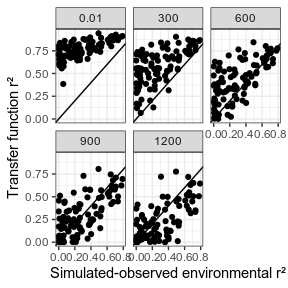Figure 5: Scatterplot of squared correlation coefficients between simulated variables and the environmental variable of interest and transfer function r2.

tibble(threshs, so_squares) |>
ggplot(aes(x = threshs, y = so_squares)) +
geom_point() +
labs(x = "h km", y = "Sum of squares")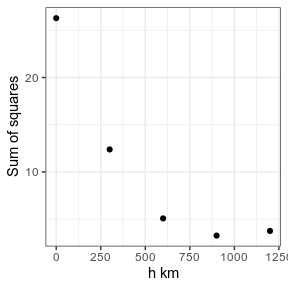Figure 6: Relationship between the sum of squares between the two r2 as function of distance h.

Following the rule described in Trachsel and Telford the value of h is estimated as h = 900 km.

Hence the three methods proposed result in similar values of h: h = 761 km for the spatially independent test set, h = 794 km for the variogram range method and h = 900 km for the variance explained method.